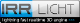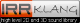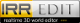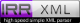Retour
Version Originale

#### ./aip/1.8aipmod/include/triangle3d.h :


// Copyright (C) 2002-2011 Nikolaus Gebhardt

// This file is part of the "Irrlicht Engine".

// For conditions of distribution and use, see copyright notice in irrlicht.h

#ifndef __IRR_TRIANGLE_3D_H_INCLUDED__
#define __IRR_TRIANGLE_3D_H_INCLUDED__

#include "vector3d.h"
#include "line3d.h"
#include "plane3d.h"
#include "aabbox3d.h"

namespace irr
{
namespace core
{

//! 3d triangle template class for doing collision detection and other things.

template <class T>
class triangle3d
{
public:

//! Constructor for an all 0 triangle

triangle3d() {}
//! Constructor for triangle with given three vertices

triangle3d(vector3d<T> v1, vector3d<T> v2, vector3d<T> v3) : pointA(v1), pointB(v2), pointC(v3) {}

//! Equality operator

bool operator==(const triangle3d<T>& other) const
{
return other.pointA==pointA && other.pointB==pointB && other.pointC==pointC;
}

//! Inequality operator

bool operator!=(const triangle3d<T>& other) const
{
return !(*this==other);
}

//! Determines if the triangle is totally inside a bounding box.

/** \param box Box to check.
\return True if triangle is within the box, otherwise false. */
bool isTotalInsideBox(const aabbox3d<T>& box) const
{
return (box.isPointInside(pointA) &&
box.isPointInside(pointB) &&
box.isPointInside(pointC));
}

//! Determines if the triangle is totally outside a bounding box.

/** \param box Box to check.
\return True if triangle is outside the box, otherwise false. */
bool isTotalOutsideBox(const aabbox3d<T>& box) const
{
return ((pointA.X > box.MaxEdge.X && pointB.X > box.MaxEdge.X && pointC.X > box.MaxEdge.X) ||

(pointA.Y > box.MaxEdge.Y && pointB.Y > box.MaxEdge.Y && pointC.Y > box.MaxEdge.Y) ||
(pointA.Z > box.MaxEdge.Z && pointB.Z > box.MaxEdge.Z && pointC.Z > box.MaxEdge.Z) ||
(pointA.X < box.MinEdge.X && pointB.X < box.MinEdge.X && pointC.X < box.MinEdge.X) ||
(pointA.Y < box.MinEdge.Y && pointB.Y < box.MinEdge.Y && pointC.Y < box.MinEdge.Y) ||
(pointA.Z < box.MinEdge.Z && pointB.Z < box.MinEdge.Z && pointC.Z < box.MinEdge.Z));
}

//! Get the closest point on a triangle to a point on the same plane.

/** \param p Point which must be on the same plane as the triangle.
\return The closest point of the triangle */
core::vector3d<T> closestPointOnTriangle(const core::vector3d<T>& p) const
{
const core::vector3d<T> rab = line3d<T>(pointA, pointB).getClosestPoint(p);
const core::vector3d<T> rbc = line3d<T>(pointB, pointC).getClosestPoint(p);
const core::vector3d<T> rca = line3d<T>(pointC, pointA).getClosestPoint(p);

const T d1 = rab.getDistanceFrom(p);
const T d2 = rbc.getDistanceFrom(p);
const T d3 = rca.getDistanceFrom(p);

if (d1 < d2)
return d1 < d3 ? rab : rca;

return d2 < d3 ? rbc : rca;
}

//! Check if a point is inside the triangle

/** \param p Point to test. Assumes that this point is already
on the plane of the triangle.
\return True if the point is inside the triangle, otherwise false. */
bool isPointInside(const vector3d<T>& p) const
{
return (isOnSameSide(p, pointA, pointB, pointC) &&
isOnSameSide(p, pointB, pointA, pointC) &&
isOnSameSide(p, pointC, pointA, pointB));
}

//! Check if a point is inside the triangle.

/** This method is an implementation of the example used in a
paper by Kasper Fauerby original written by Keidy from
Mr-Gamemaker.
\param p Point to test. Assumes that this point is already
on the plane of the triangle.
\return True if point is inside the triangle, otherwise false. */
bool isPointInsideFast(const vector3d<T>& p) const
{
const vector3d<T> f = pointB - pointA;
const vector3d<T> g = pointC - pointA;

const f32 a = f.dotProduct(f);
const f32 b = f.dotProduct(g);
const f32 c = g.dotProduct(g);

const vector3d<T> vp = p - pointA;
const f32 d = vp.dotProduct(f);
const f32 e = vp.dotProduct(g);

f32 x = (d*c)-(e*b);
f32 y = (e*a)-(d*b);
const f32 ac_bb = (a*c)-(b*b);
f32 z = x+y-ac_bb;

// return sign(z) && !(sign(x)||sign(y))

return (( (IR(z)) & ~((IR(x))|(IR(y))) ) & 0x80000000)!=0;
}

//! Get an intersection with a 3d line.

/** \param line Line to intersect with.
\param outIntersection Place to store the intersection point, if there is one.
\return True if there was an intersection, false if not. */
bool getIntersectionWithLimitedLine(const line3d<T>& line,
vector3d<T>& outIntersection) const
{
return getIntersectionWithLine(line.start,
line.getVector(), outIntersection) &&
outIntersection.isBetweenPoints(line.start, line.end);
}

//! Get an intersection with a 3d line.

/** Please note that also points are returned as intersection which
are on the line, but not between the start and end point of the line.
If you want the returned point be between start and end
use getIntersectionWithLimitedLine().
\param linePoint Point of the line to intersect with.
\param lineVect Vector of the line to intersect with.
\param outIntersection Place to store the intersection point, if there is one.
\return True if there was an intersection, false if there was not. */
bool getIntersectionWithLine(const vector3d<T>& linePoint,
const vector3d<T>& lineVect, vector3d<T>& outIntersection) const
{
if (getIntersectionOfPlaneWithLine(linePoint, lineVect, outIntersection))
return isPointInside(outIntersection);

return false;
}

//! Calculates the intersection between a 3d line and the plane the triangle is on.

/** \param lineVect Vector of the line to intersect with.
\param linePoint Point of the line to intersect with.
\param outIntersection Place to store the intersection point, if there is one.
\return True if there was an intersection, else false. */
bool getIntersectionOfPlaneWithLine(const vector3d<T>& linePoint,
const vector3d<T>& lineVect, vector3d<T>& outIntersection) const
{
const vector3d<T> normal = getNormal().normalize();
T t2;

if ( core::iszero ( t2 = normal.dotProduct(lineVect) ) )
return false;

T d = pointA.dotProduct(normal);
T t = -(normal.dotProduct(linePoint) - d) / t2;
outIntersection = linePoint + (lineVect * t);
return true;
}

//! Get the normal of the triangle.

/** Please note: The normal is not always normalized. */
vector3d<T> getNormal() const
{
return (pointB - pointA).crossProduct(pointC - pointA);
}

//! Test if the triangle would be front or backfacing from any point.

/** Thus, this method assumes a camera position from which the
triangle is definitely visible when looking at the given direction.
Do not use this method with points as it will give wrong results!
\param lookDirection Look direction.
\return True if the plane is front facing and false if it is backfacing. */
bool isFrontFacing(const vector3d<T>& lookDirection) const
{
const vector3d<T> n = getNormal().normalize();
const f32 d = (f32)n.dotProduct(lookDirection);
return F32_LOWER_EQUAL_0(d);
}

//! Get the plane of this triangle.

plane3d<T> getPlane() const
{
return plane3d<T>(pointA, pointB, pointC);
}

//! Get the area of the triangle

T getArea() const
{
return (pointB - pointA).crossProduct(pointC - pointA).getLength() * 0.5f;

}

//! sets the triangle's points

void set(const core::vector3d<T>& a, const core::vector3d<T>& b, const core::vector3d<T>& c)
{
pointA = a;
pointB = b;
pointC = c;
}

//! the three points of the triangle

vector3d<T> pointA;
vector3d<T> pointB;
vector3d<T> pointC;

private:
bool isOnSameSide(const vector3d<T>& p1, const vector3d<T>& p2,
const vector3d<T>& a, const vector3d<T>& b) const
{
vector3d<T> bminusa = b - a;
vector3d<T> cp1 = bminusa.crossProduct(p1 - a);
vector3d<T> cp2 = bminusa.crossProduct(p2 - a);
return (cp1.dotProduct(cp2) >= 0.0f);
}
};

//! Typedef for a f32 3d triangle.

typedef triangle3d<f32> triangle3df;

//! Typedef for an integer 3d triangle.

typedef triangle3d<s32> triangle3di;

} // end namespace core

} // end namespace irr

#endif


Correctionsxhtml 1.0
css 2.1
Propulsé par FluxBB